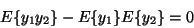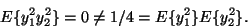Next: Why Gaussian variables are Up: What is independence? Previous: Definition and fundamental properties

## Uncorrelated variables are only partly independent

A weaker form of independence is uncorrelatedness. Two random variablesand y2 are said to be uncorrelated, if their covariance is zero:(10)

If the variables are independent, they are uncorrelated, which follows directly from Eq. (11), taking h1(y1)=y1 and h2(y2)=y2.

On the other hand, uncorrelatedness does not imply independence. For example, assume that (y1,y2) are discrete valued and follow such a distribution that the pair are with probability 1/4 equal to any of the following values: (0,1),(0,-1),(1,0),(-1,0). Then y1 and y2 are uncorrelated, as can be simply calculated. On the other hand,(11)

so the condition in Eq. (11) is violated, and the variables cannot be independent.

Since independence implies uncorrelatedness, many ICA methods constrain the estimation procedure so that it always gives uncorrelated estimates of the independent components. This reduces the number of free parameters, and simplifies the problem.Next: Why Gaussian variables are Up: What is independence? Previous: Definition and fundamental properties
Aapo Hyvarinen
2000-04-19